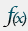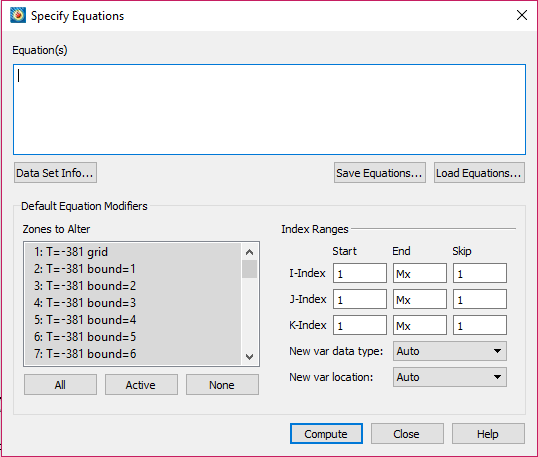# Calculating a New Variable

## Data Alteration using Equations to Calculate a New Variable

Engineers using Tecplot 360 often need to create a new variable which is based on a numeric relationship of existing variables already loaded into Tecplot.

This powerful capability for calculating a new variable uses a simple method. To start, load your data into Tecplot 360. In this example, we loaded the VortexShedding.plt data located in the Tecplot 360 examples folder.

Choose Alter -> Specify Equations from the Data menu.
Alternately, click the equations iconYou will see the Specify Equations dialog shown at right.

We will now calculate the difference between two variables. In the Zones to Alter list, click All.

Initialize the new variable T(K)Difference, by typing in the Equation(s) window:

{T(K)Difference} = 0

Click Compute

Now find the difference for variable T(K) between zone 2 and 3 (for example, T(K) in zone 3 – T(K) in zone 2) to T(K)Difference). You can do this for any two variables that have a similar structure.

Select the zones you want to receive the difference value. Type the following equation using the exact variable name from the Data Set Information dialog.

{T(K)Difference} = {T(K)}-{T(K)}

Click Compute

The new variable T(K)Difference is now available for plotting. Open the Data Set Information dialog from the Data menu and view the new variable T(K)Difference.

Note that changes made to the dataset in the Specify Equations dialog are not made to the original data file. You can save the changes by saving a layout file or writing the new data to a file. Saving a layout file will keep your data file in its original state, but use journaled commands to reapply the equations.Learn more in Chapters 20 and 21 of the Tecplot 360 User Manual.

Tecplot 360 User Manual

### Related Video Tutorials

This blog was originally published in 2013 and has been updated and expanded.